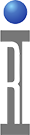DRAFT: This document is still being modified by the author. Any information here is subject to change.

When testing 2nd and 3rd harmonics of a part driven to relatively high output levels, it is sometimes difficult to see the harmonics contributed by the Device Under Test (DUT) because the test equipment is adding harmonics of its own. In the extreme case, where the part produces almost no harmonic power at all, the measurement at the 2nd or 3rd harmonic is entirely due to signals generated in the tester itself.

In one example, a part driven to +15 dBm when operating normally had harmonic power better than -80 dBc. But measurement on a tester showed the harmonic power to be about -60 dBc. This indicated the floor of the harmonic measurement capability of the tester under the driving conditions and the choices made for its receiver attenuation and IF gain.

Measurement Technique

To see below this "harmonic floor" of the tester, a novel method was developed. This method exploits the nature of RF measurements in which different signals add together as phasors (or vectors) with varying phase relationships as measurement conditions, such as stimulus frequency, are changed. (See figure below.) For demonstration purposes, assume you have two harmonic sources, the DUT and the test equipment itself. The two signals add together at the receiver as vectors. The absolute magnitude of the resulting signal will vary according to the phase relationship between the two signals.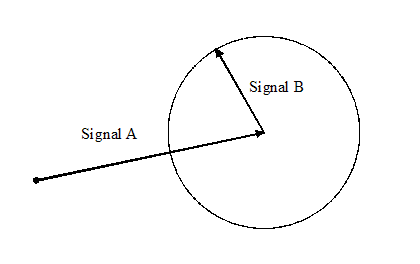Take the same two signals, but now change the stimulus frequency very slightly. The phase relationship of the signals changes according to the relative path lengths of the two sources of harmonics. As you continue to change the stimulus frequency, the smaller signal will eventually describe a complete circle around the larger signal. Looking at the result as a graph of detected signal magnitude versus frequency, the addition of the rotating vectors describes a sinusoidal wave. The average value of the sinusoid is due to the larger signal and the peak-to-peak amplitude of the sinusoid is due to the smaller signal. Thus, you can separate the two sources of harmonics by sweeping the frequency of the stimulus and calculating the peak-to-peak and average values of the resulting waveform.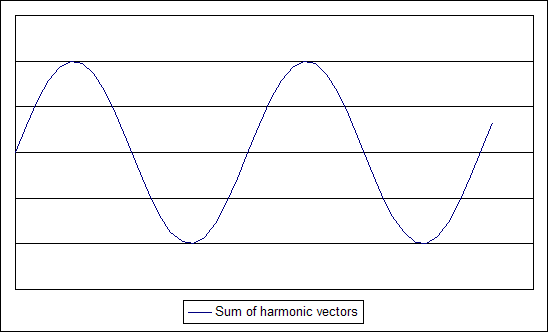There are considerations when using this technique. While it is straightforward to separate the two signals using the method described, it does not identify which signal is the "DUT" and which is the "tester" component. It is up to the test engineer to arrange limits in such a way to catch devices which fail. The technique is not able to determine if the smaller harmonic is due to the DUT or another harmonic signal generated in the test system. In practice, this measurement has been shown to extend the range of measurement from -60 dBc to -70 dBc or so, but some interpretation of the results is needed.

Example Testplan

In the testplan fragment below, we demonstrate how to efficiently measure a small set of frequencies around a second harmonic and calculate the average and peak components of the overall measured response.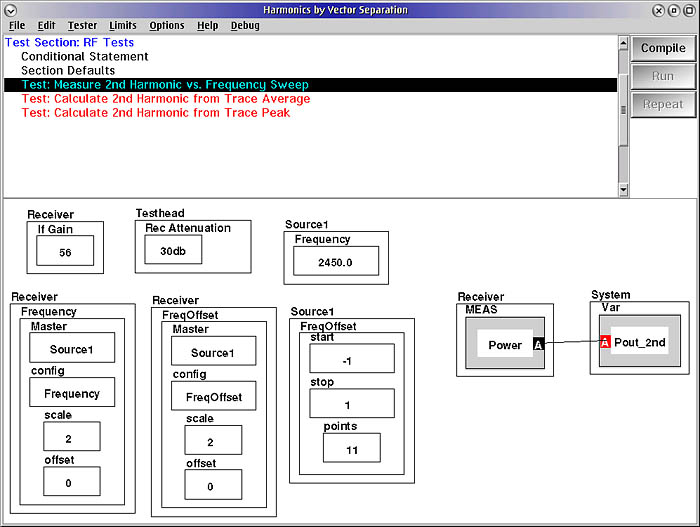First the receiver is used to measure a set of frequencies, in this case 11 points, around a harmonic of interest. Source 1 is set to its fundamental frequency, in this case 2450 MHz. The frequency of the receiver's local oscillator is slaved to the source frequency multiplied by two (and with no offset). This causes the receiver's measurement frequency to track the 2nd harmonic of the Source 1 frequency.

The two sources of 2nd harmonics we are measuring are physically separated by a great distance. Therefore, the frequency of the sinusoidal signal we are measuring is very rapidly changing with frequency shifts. In our example, we can get an adequate number of samples across the complete sinusoid by measuring 11 points between -1 MHz to +1 MHz relative to the fundamental tone. (This means the receiver L.O. will move -2 MHz to +2 MHz over the same 11 points, again because it is slaved to Source 1 with a multiplier of two.)

The power of the 2nd harmonic is measured and saved as a linear value (Watts) rather than a log value (dBm). The calculations which follow must be done in linear terms. The results are converted to dBm after the calculations.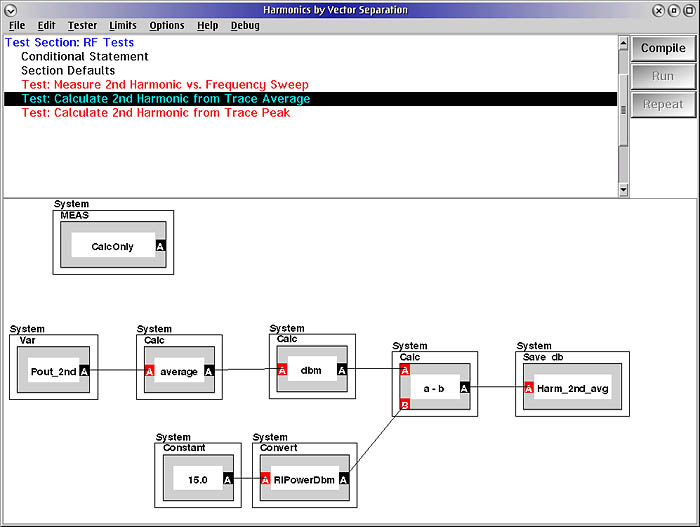To compute the average value of the 2nd harmonic power, the entire array of 11 values is averaged together. Because a measurement relative to the fundamental is required, the known power at the fundamental tone is subtracted (after conversion to dBm). The result is stored as a log value relative to the carrier (dBc).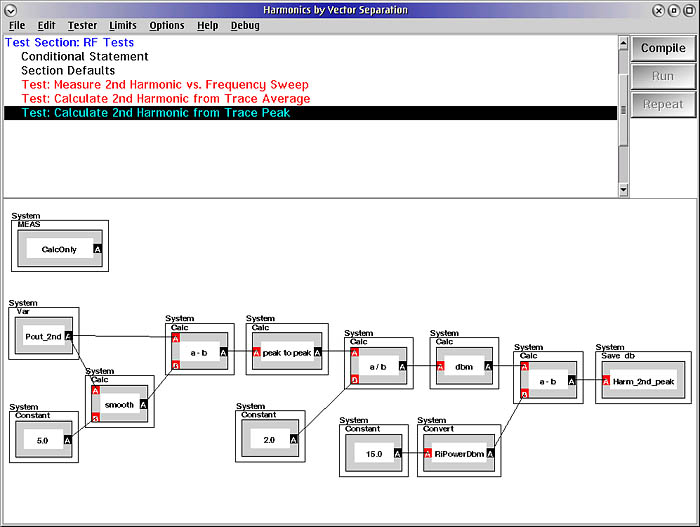Finding the peak variation is only a bit more complicated. To remove any slope or low-frequency variations from the signal we are calculating, we first apply a "smoothing" function to the measurement points. This does a moving average with 5 points on either side of the center value. This "low pass filtered" version of the waveform is subtracted from the measured data to leave only the fast-moving sinusoid we are interested in.

After high-pass filtering the waveform, the peak-to-peak value is calculated and the result divided by two to get the peak value. The peak value is converted to dBm and made relative to the carrier which is known to be 15 dBm. The result is stored as the "peak" 2nd harmonic value.

Results

...To be continued...

https://roos.com/docs/JBAT-6YR8XS
ROOS INSTRUMENTS CONFIDENTIAL AND PROPRIETARY